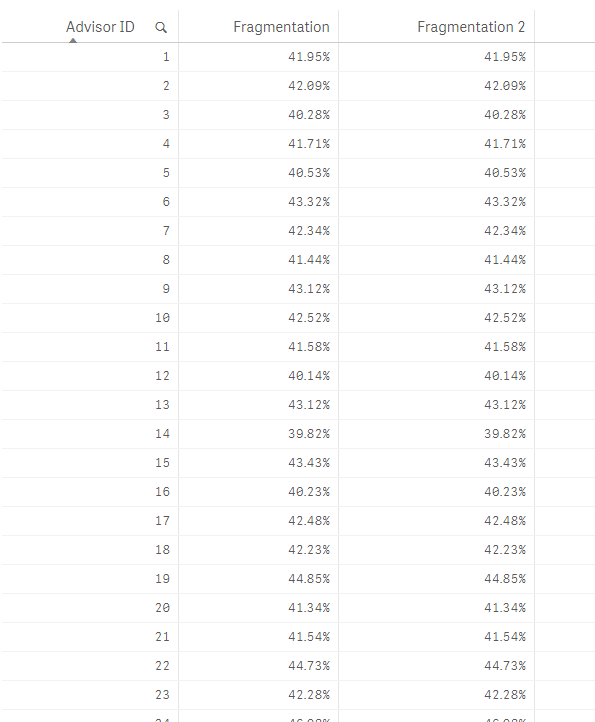# App Development

Announcements
April 22, 2PM EST: Learn about GeoOperations in Qlik Sense SaaS READ MORE
cancel
Showing results for
Did you mean:Partner

## Counting securities associated with 3 or less accounts

Hi,

I am trying to count securities (dimension) that are associated with 3 or less accounts (another dimension). Both IDs appear in my fact table. I need the amount per advisor ID, a third dimension, also in the fact table. The following query actually works but is really slow:

sum(aggr({<[Snapshot Date] = {"\$(=max([Snapshot Date]))"}>}if(count(distinct [Account ID]) <= 3, 1, 0),[Security ID], [Advisor ID]))

I guess the issue is the double aggregation in my query. Any idea on how I could get the same result a little faster?

Thanks!

1 Solution

Accepted SolutionsMVP

Try this expression:

Count(DISTINCT {<Key = {"=Count(DISTINCT [Account ID]) <= 3"}, [Snapshot Date] = {"\$(=Max([Snapshot Date]))"}>} Key)

/Count({<[Snapshot Date] = {"\$(=Max([Snapshot Date]))"}>} Distinct [Security ID])9 RepliesMVP

Would you be able to share a small sample? Would be easier to resolve if we can see the issuePartner
Author

Hi again,

I can barely reproduce the slowness in a small scale sample. I generated a 1M rows random dataset to try to mimic the issue. Mine contains many million rows. At least you will get a better idea of what I am trying to do.

Thanks!MVP

How about something like this in the script:

[Position Monthly]:

[ID],

[Snapshot Date],

[Account ID],

[Security ID]

FROM [lib://Temp/fragmentation_sample.xlsx]

(ooxml, embedded labels, table is [Position Monthly]);

AggrTable:

If(Count(DISTINCT [Account ID]) <= 3, 1, 0) as Flag

Resident [Position Monthly]

Group By [Security ID], [Advisor ID];

New Expression:

Sum({<[Snapshot Date] = {"\$(=Max([Snapshot Date]))"}>} Flag)/Count({<[Snapshot Date] = {"\$(=Max([Snapshot Date]))"}>} Distinct [Security ID])Partner
Author

The thing is the accounts can be filtered, so when the filter is applied, the number of fragmented securities will changeMVP

Another option would be something like this:

[Position Monthly]:

[ID],

[Snapshot Date],

[Account ID],

[Security ID]

FROM [lib://Temp/fragmentation_sample.xlsx]

(ooxml, embedded labels, table is [Position Monthly]);

Expression:

Sum({<Key = {"=Count(DISTINCT [Account ID] <= 3"}, [Snapshot Date] = {"\$(=Max([Snapshot Date]))"}>} 1)/Count({<[Snapshot Date] = {"\$(=Max([Snapshot Date]))"}>} Distinct [Security ID])Partner
Author

Hi,

I tried your solution in my sample app, and I didn't get it to work. Here is the modified sample.

I am not sure I understand the concept neither... If I use an autonumber, I understand that it will create in memory a temporary table with autoincrement keys for each Advisor ID / Security combination. How can these keys match {"=Count(DISTINCT [Account ID] <= 3"}?MVP

Try this expression:

Count(DISTINCT {<Key = {"=Count(DISTINCT [Account ID]) <= 3"}, [Snapshot Date] = {"\$(=Max([Snapshot Date]))"}>} Key)

/Count({<[Snapshot Date] = {"\$(=Max([Snapshot Date]))"}>} Distinct [Security ID])Partner
Author

Indeed! Thanks! Would you mind to explain me why this is working? I don't get the key idea...MVP

I am creating a key where we are checking the count of account. To use set analysis in this scenario, we need to use a search string (=Count(DISTINCT [Account ID]) <= 3) but this will only work against a unique combination of Security ID and Advisor ID (This is based on the fact that you were using the if statement and count of account on these two dimensions in the aggr function. This gave me the idea to combine these to to form a key to use in set analysis). Once combined, I had to play around with it a little to get the matching number.

I hope all the above made sense? I would be interested in knowing if it actually improved performance or if it is almost the same as before?

Best,

SunnyTags
Community Browser